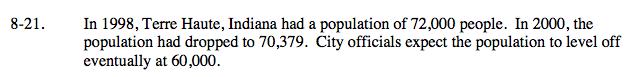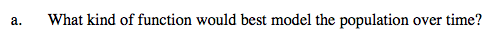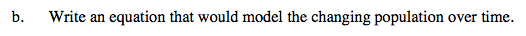### Home > A2C > Chapter 8 > Lesson 8.1.2 > Problem8-21

8-21.Which functions level off eventually?

An exponential function with an asymptote at y = 60,000 would be the best model.$72000 = a(b)^0 + 60000$

$72000 = a + 60000$

$12000 = a$

$b \approx 0.93$

$70379 = a(b)^0 + 60000$

$10379 = 12000(b)^2$

$\frac{10379}{12000} =\ b^2$

$y = 12000(0.93)^t + 60000$